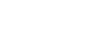# Physics Paper 3 Questions and Answers with Confidential - Maranda Post Mocks 2020/2021

Question one

You are provided with the following:

• 2 new dry cells size
• A cell holder
• A switch
• A milliammeter of range 0 to 1 mA
• A capacitor labeled C
• 8 connecting wires, at least four with crocodile clips on one end
• A stopwatch
• A carbon resistor labeled R

Proceed as follows

1. Connect the circuit as shown in the Figure 1 below, where P and Q are crocodile clips.2. Close the switch S
3. Name the process which takes place when the switch S is closed (1 mark)
4. Connect the crocodile clips P and Q. Observe and record the highest reading of the milliammeter 1. (This is the current at to=0)
Io = ....... mA (1 mark)
5. While the milliammeter show the maximum value of current Io, open the switch S and start the stop watch simultaneously. Stop the stop watch when the current has dropped from Io to 0.5 mA. Read and record in the table below the time taken.
6. Reset the stop watch and close the switch. Repeat the procedure in (e) to measure and record the time taken for the current to drop from Io to each of the other values shown in the Table 1 below. (4marks)
 Current I(MA) 0.5 0.4 0.3 0.2 0.1 Time t(s)
7. Plot a graph of Current I (y-axis)(mA) against time t(s) (4marks)
8. From your graph, find W the value of I when t= 10s. (2marks)
9. Given that A = 10W, determine the value of A. (1mark)
10. Determine the voltage across R at t = 10s given that R=4.7kΩ (3marks)

Question 2

PART A

You are provided with the following apparatus;

• A nichrome wire
• A 50g mass
• A metre rule
• A test-tube
• A retort stand, boss and clamp

Proceed as follows.

1. Measure the length, L, of the nichrome wire provided (1mark)
2. Wind the whole length of the wire tightly on the test-tube making sure that the turns are as close as possible but not overlapping. Measure the length, φ, of the coil made.(1mark)
3. Remove the coil from the test-tube. Straighten the first and the last turns of coil. Bend one end to make a hook.
4. Count and record the number, N, of complete turns remaining on the coil.
5. Measure and record the distance h1, between the end turns of the coil as shown on the Figure 2 below. (1 mark)6. Load a 50 g mass on the coil as shown in Figure 3 above. Measure and record the distance, h2, between the end turns of the coil. (1 mark)
7. Determine the spring constant K in S.I units. (2 marks)
8. Obtain the constant, p, for the wire from the expression: (3marks)
P = 4mgR3
Kr4
Where: m is the mass used, g is acceleration due to gravity (g =10m/s2 ), and
R =
2ΠN
and
r = φ
2N

PART B

You are provided with the following apparatus:

• A screen with cross wire
• lens and a lens holder
• A white screen
• A Metre rule
• Lighting source. (match box)

Proceed as follows:

1. Arrange the lens and screen as shown in the diagram below.Adjust the distance of the lens from the screen until a sharp image of a distant object is formed on the screen. Measure and record the distance d (1mark)
2. Place the metre rule on a horizontal table so that the millimeter scale faces upwards, place the candle at one end of the metre rule and the screen with cross wire at the zero cm mark (just near the candle). Arrange the lens and the white screen as shown below:1. Adjust the lens so that the distance x is 30cm.
2. Adjust also the position of the white screen to obtain a sharp image of the cross wire and record the value of y in the table below.
3. Repeat steps b (i) and b (ii) above for values of x = 35cm, 40cm,45cm, 50cm and record the corresponding values of y each time in the table below.
4. Hence complete the table.
 x(cm) 30 35 40 45 50 y(cm) y + x (cm) yx (cm2)
5. Plot a graph of (y + x)(y-axis) against yx.(x-axis) (4marks)
4. Given that the equation of graph isUse the graph to find the value of f. (3 marks)## Confidential

Question 1

• A metre rule
• A vernier calliper
• 50g mass and 100g mass
• water in a beaker
• A beaker
• knife edge
• tissue paper at least 30 cm long

Question 2

• Two new dry cells
• an ammeter 0-1A
• a voltmeter 0-5A
• A resister wire labelled XY on a mm scale
• Jockey or crocodile clip
• Cell holder
• Switch
• six connecting wires
• micrometer screw gauge## Marking Scheme• ✔ To read offline at any time.
• ✔ To Print at your convenience
• ✔ Share Easily with Friends / Students